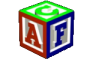EE Shader global matrix equivalents Author Message
3DRaddictMemberPost: #1 EE Shader global matrix equivalents What would help me immensely in my understanding of the EE shader code would be if you could kindly supply me with the names of the EE equivalents for these global matrices used in most HLSL shaders: WorldMatrix ViewMatrix ViewProjectionMatrix ViewInverseTransformMatrix
09-16-2014 03:21 PM
EsenthelPost: #2 RE: EE Shader global matrix equivalents Hi, Please look into: "Constants.h" Code: ```Matrix4 MatrixProj                    ; // projection  matrix    Matrix  MatrixCam                     ; // camera      matrix    #define MatrixOC0  MatrixOC  // object camera matrix          (use this for no skinning) (this is object matrix * inversed camera matrix)``` instead of using them directly, I recommend using following functions: Code: ```pos=          TransformPos(vtx.pos4()); nrm=Normalize(TransformDir(vtx.nrm ())); outVtx=Project(pos);``` For full example you can look at "Blend.cpp" shader, and the vertex shader: Code: ```void VS (    VtxInput vtx, ..```
fatcoder